GMAT Math : DSQ: Understanding real numbers

Example Questions

1 2 4 Next →

Example Question #1260 : Data Sufficiency Questions

Define an operation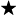on the real numbers as follows:

If bothandare both positive, then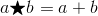.

If bothandare not both positive, then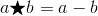.

Evaluate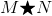.

Statement 1: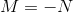.

Statement 2: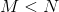.

BOTH statements TOGETHER are insufficient to answer the question.

Statement 1 ALONE is sufficient to answer the question, but Statement 2 ALONE is NOT sufficient to answer the question.

BOTH statements TOGETHER are sufficient to answer the question, but NEITHER statement ALONE is sufficient to answer the question.

EITHER statement ALONE is sufficient to answer the question.

Statement 2 ALONE is sufficient to answer the question, but Statement 1 ALONE is NOT sufficient to answer the question.

BOTH statements TOGETHER are insufficient to answer the question.

Explanation:

Assume both statements to be true. From Statement 1,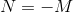, soandare each other's opposites. Either one is positive and one is negative, or both are equal to 0; either way, the definition of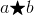forandnot both positive is used, and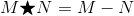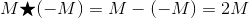Statement 2 tells us nothing except that, so it can only be deduced thatis negative, and so is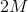With no further information,cannot be evaluated.

1 2 4 Next →

Tired of practice problems?

Try live online GMAT prep today.